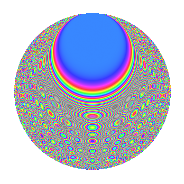# Properties

 Label 430.2.rLevel 430 Weight 2 Character orbit r Rep. character $$\chi_{430}(27,\cdot)$$ Character field $$\Q(\zeta_{28})$$ Dimension 264 Newform subspaces 1 Sturm bound 132 Trace bound 0

# Related objects

## Defining parameters

 Level: $$N$$ = $$430 = 2 \cdot 5 \cdot 43$$ Weight: $$k$$ = $$2$$ Character orbit: $$[\chi]$$ = 430.r (of order $$28$$ and degree $$12$$) Character conductor: $$\operatorname{cond}(\chi)$$ = $$215$$ Character field: $$\Q(\zeta_{28})$$ Newform subspaces: $$1$$ Sturm bound: $$132$$ Trace bound: $$0$$

## Dimensions

The following table gives the dimensions of various subspaces of $$M_{2}(430, [\chi])$$.

Total New Old
Modular forms 840 264 576
Cusp forms 744 264 480
Eisenstein series 96 0 96

## Trace form

 $$264q - 8q^{6} + O(q^{10})$$ $$264q - 8q^{6} - 40q^{13} + 44q^{16} - 8q^{17} - 16q^{21} - 24q^{23} - 8q^{25} + 48q^{31} - 56q^{33} + 32q^{35} + 256q^{36} - 100q^{38} + 40q^{41} - 168q^{43} + 48q^{47} - 280q^{51} - 16q^{52} + 24q^{53} - 8q^{56} - 40q^{57} - 16q^{60} - 84q^{62} + 84q^{65} + 144q^{66} + 40q^{67} + 20q^{68} - 252q^{73} - 56q^{77} - 56q^{78} + 48q^{81} - 56q^{82} - 44q^{83} + 20q^{86} - 144q^{87} + 4q^{90} + 24q^{92} + 28q^{95} + 8q^{96} - 60q^{97} + O(q^{100})$$

## Decomposition of $$S_{2}^{\mathrm{new}}(430, [\chi])$$ into newform subspaces

Label Dim. $$A$$ Field CM Traces $q$-expansion
$$a_2$$ $$a_3$$ $$a_5$$ $$a_7$$
430.2.r.a $$264$$ $$3.434$$ None $$0$$ $$0$$ $$0$$ $$0$$

## Decomposition of $$S_{2}^{\mathrm{old}}(430, [\chi])$$ into lower level spaces

$$S_{2}^{\mathrm{old}}(430, [\chi]) \cong$$ $$S_{2}^{\mathrm{new}}(215, [\chi])$$$$^{\oplus 2}$$

## Hecke Characteristic Polynomials

There are no characteristic polynomials of Hecke operators in the database